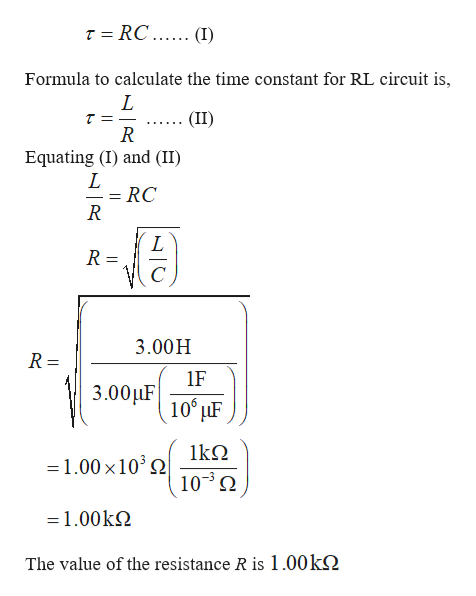# An RL circuit with L = 3.00 H and an RC circuit withC = 3.00 µF have the same time constant. If the two circuitshave the same resistance R, (a) what is the value of R and (b)what is this common time constant?

Question
9 views

An RL circuit with L = 3.00 H and an RC circuit with
C = 3.00 µF have the same time constant. If the two circuits
have the same resistance R, (a) what is the value of R and (b)
what is this common time constant?

check_circle

Step 1

Formula to calculate the value of...help_outlineImage TranscriptioncloseT = RC ..... (I) Formula to calculate the time constant for RL circuit is, (II) R Equating (I) and (II) = RC R = 3.00H R = 1F 3.00µF 10° µF 1kΩ =1.00 x10° 2 10° N =1.00kN The value of the resistance R is 1.00k2 fullscreen

### Want to see the full answer?

See Solution

#### Want to see this answer and more?

Solutions are written by subject experts who are available 24/7. Questions are typically answered within 1 hour.*

See Solution
*Response times may vary by subject and question.
Tagged in

### Other